## Introduction

We begin counting objects from 1, 2, 3, 4, etc. These numbers are called natural numbers. Whole numbers start from ‘0’, i.e., 0, 1, 2, 3, and so on.

Which of the following temperatures are below 0° on this thermometer?The temperatures -10° and-20° are below 0°.

What are these numbers called? These numbers are called negative numbers. Negative numbers are numbers with a negative sign. Also, negative numbers are less than zero.

Integers are a set of numbers which include positive numbers, negative numbers, and zero

#### Concepts

The chapter ‘Integers’ covers the following concepts:

#### Understanding Integers

You know that integers are a set of numbers which include positive numbers, negative numbers, and zero.So, – 1, – 2, – 3, – 4, ... which are called negative numbers are the negative integers and 1, 2, 3, 4, ... which are called positive numbers are the positive integers.

Watch the video below to understand why we need integers:

When do we use integers? Integers are used when we need to represent numbers with a negative sign. Integers are also used to represent two opposite or contradicting situations. For example, the opposite of a loss of ₹500 is a profit of ₹500.

The table below shows some contradicting situations: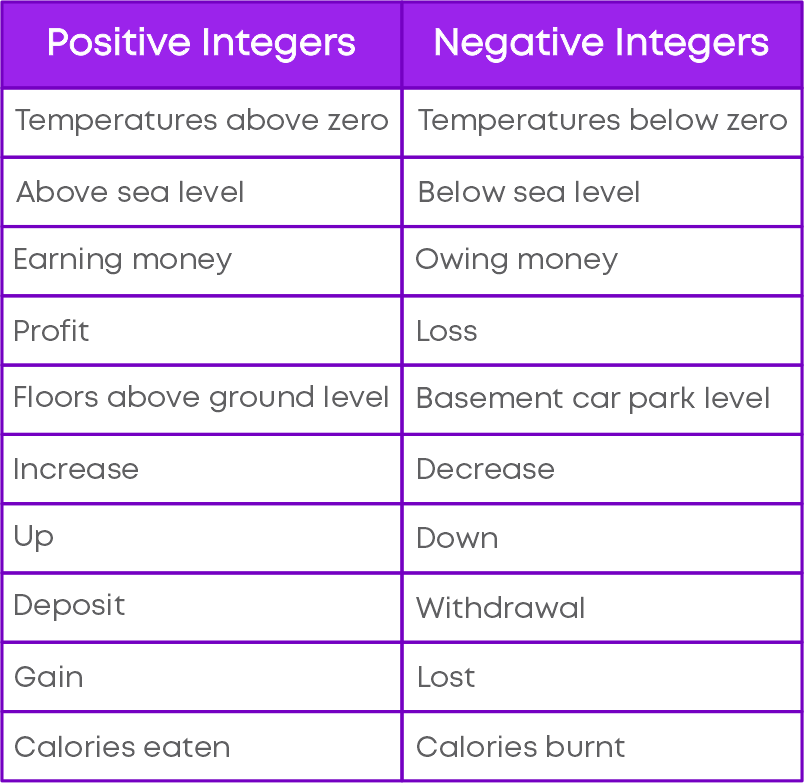A deposit of rupees two hundred.

• First, identify the keyword

Here, the keyword is deposit. Deposit means putting money into an account. We take this as a positive integer.

.
• Write the number given in the context with an appropriate sign

Thus, we can represent “A deposit of rupees two hundred” as + 200.

Now, let us take another context:

Withdrawal of rupees three hundred.

Here the keyword is withdrawal. Withdrawal means we are taking money out of an account. We should take this as a negative integer. Hence, we can represent “Withdrawal of rupees three hundred” as (- 300).

Represent Integers On A Number Line

Now, let us understand how to locate an integer on the number line. Follow these steps:

• To form a number line, draw a horizontal straight line and mark points on it at equal distances.
• Mark a suitable point as ‘0’ and mark positive integers 1, 2, 3, …. to the right of ‘0’ and mark negative integers -1, -2, -3, … to the left of ‘0’.
• If you are marking positive numbers move to the right of zero.
• If you are marking negative numbers move to the left of zero.

For example, to mark -3 on the number line, follow the steps above to get: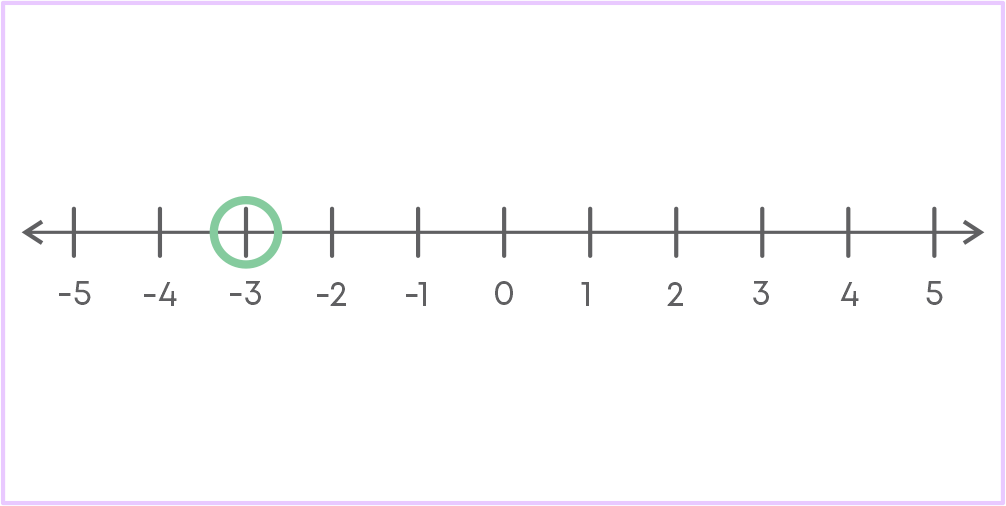Successor/ Predecessor Of An Integer

The successor of an integer is an integer which is one step to the right of the integer. For example, the successor of integer (-1) is 0.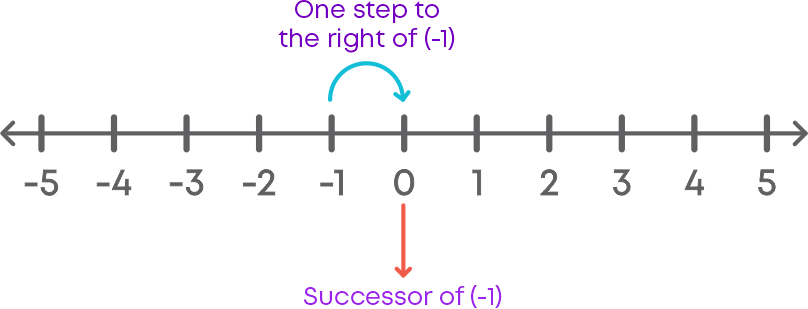The predecessor of an integer is an integer which is one step to the left of the integer. For example, the predecessor of integer (-1) is (-2).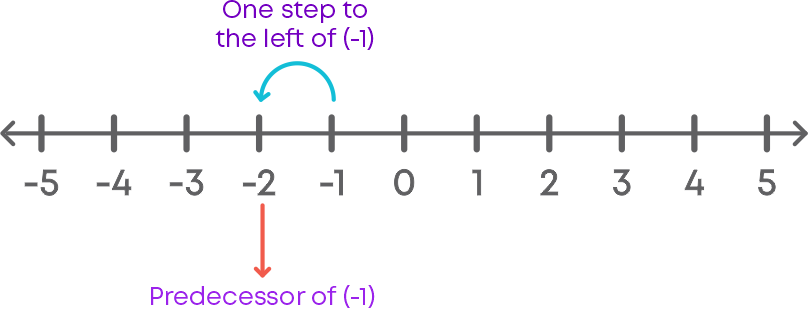Real-Life Applications Of Integers

Negative integers come in handy when a driver must slow down speed or decelerate. For example, a person is driving a car at the speed of 70 kmph on the highway. After the highway ends, the speed limit drops to 40 kmph, so the driver decelerates by 30 kmph to drive at the correct speed limit.Negative integers are also used to measure depth below sea level. For example, the Dead Sea in Israel is located at approximately 423 m below sea level. This can be represented numerically as –423 m.#### Comparing Integers

You know how to compare whole numbers. Now, let us try to compare integers; we will use a number line for the same. To compare two integers on a number line, let us see how integers are positioned on a horizontal number line.Consider the integers 1 and 4 on the number line. What do you notice?

1 is to the left of 4, hence, 1 < 4.

A number line need not always be horizontal. It can also be vertical. Look at the vertical number line given below. Here, positive numbers are above ‘0’ and negative numbers are below ‘0’.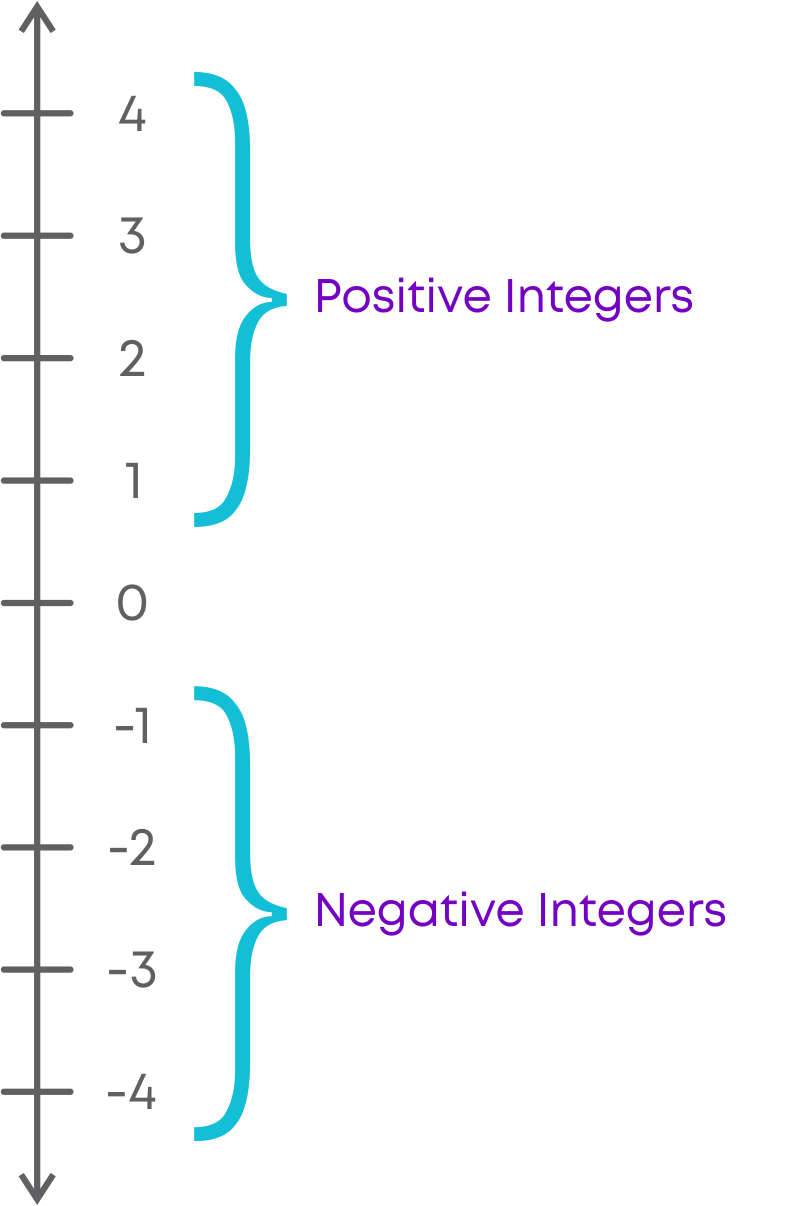On this vertical number line, consider two integers, 2 and -4.

Here, -4 is below 2, hence, -4 is less than 2.

To compare integers without a number line, remember that for positive numbers, as the digit gets bigger, the value also becomes bigger. For negative numbers, as the digit gets bigger, the value becomes smaller. For example, 6 is greater than 5. Now consider the same numbers but with a minus sign: - 5 is greater than - 6.

Now that you know how to compare integers, you can easily arrange integers in ascending or descending order.

Ascending order of integers means arranging the integers from the smallest to the greatest. Consider the integers 15, (-23), 8, (-12) and (-2). Let us arrange the integers in ascending order:

(-23) < (-12) < (-2) < 8 < 15

Descending order of integers means arranging the integers from the greatest to the smallest. Consider the integers 7, (-24), 24, (-8), (11). Let us arrange the integers in descending order:

24 > 11 > 7 > (-8) > (-24)

#### Operations on Integers

You know how to add two whole numbers on a number line. Let us understand how to use a number line to add two integers. For example, to add integers 2 and (-5), we use a number line and follow these steps:

• Draw a number line.
• Mark 2 on the number line.
• From 2, move 5 steps backwards.Hence, 2 + (-5) = (-3).

Did you know that the additive inverse of a number ‘a’ is the number that, when added to ‘a’, gives zero. This number is also called as the negative of the integer.

The opposite of a positive number is the negative of the number. So, the additive inverse of ‘a’ is (-a).

The opposite of a negative number is the positive of the number. So, the additive inverse of (-a) is ‘a’.

Now, look at these examples:

• (-5) + 3 = (-2)
• 5 + (-3) = 2
• (50) + (-30) = 20
• (-50) + 30 = (-20)

In the examples above, a positive and a negative integer is added. Do you notice a pattern in the result?

We can see that the sign of the sum depends on the numbers that are added, i.e., the sign of the result is the same as the biggest number in the sum.

Remember, when we add 2 negative integers, the sign of the result is always negative. When we add a positive integer and a negative integer, the sign of the integer is always the sign of the bigger number. Essentially, follow these rules:

If integers are of the same sign,

ADD and KEEP the SAME SIGN

If integers are of different signs,

SUBTRACT and KEEP the SIGN of the BIGGER NUMBER

Let us now learn how to subtract integers using a number line. For example, to subtract integers (-2) from (-5), we used a number line and follow these steps:

• Draw a number line.
• Mark -5 on the number line.
• From -5, move 2 steps forwards.Hence, (-5) - (-2) = (-3).

You can see that subtraction of integers is the same as adding the additive inverse. Consider two integers 'a' and 'b'. Additive inverse of 'b' is '-b'. So, a - b = a + (-b)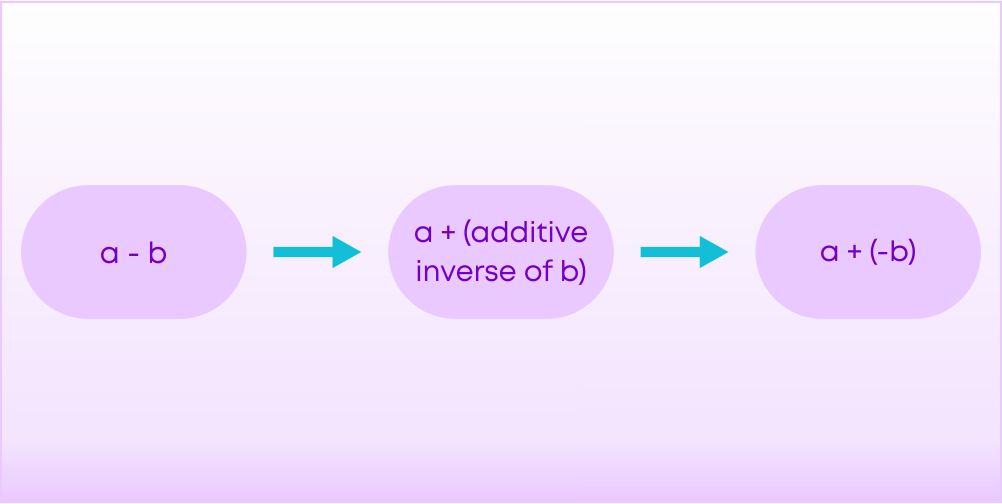#### Common Errors

The following are the topics in which students make common mistakes when dealing with integers:

• 1. The successor of an integer
• 2. Predecessor of an integer
• 3. Comparing negative integers
• 5. Subtraction of integers

#### The Successor Of An Integer

What is the successor of the integer (-3)? Is it (-2) or (-4)? The successor of an integer is an integer which is one step to the right of the integer. If we move 1 step to the right of (-3) we get the successor of (-3).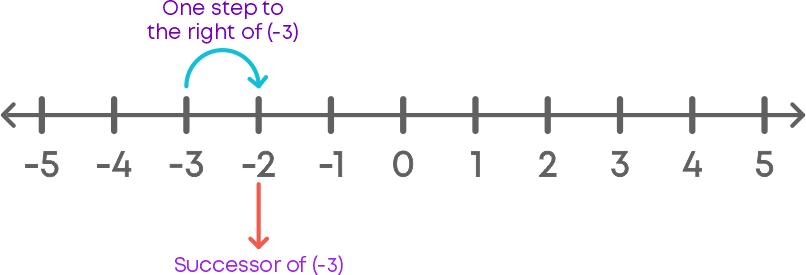Hence, the successor of integer (-3) is (-2).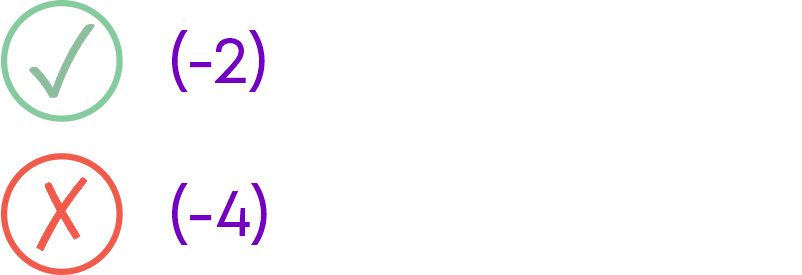#### Predecessor Of An Integer

What is the predecessor of the integer (-1)? Is it (-2) or 0? The predecessor of an integer is an integer which is one step to the left of the integer. If we move 1 step to the left of (-1) we get the predecessor of (-2).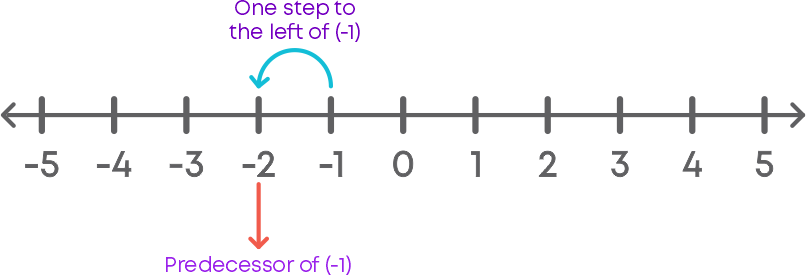Hence, the predecessor of the integer (-1) is (-2).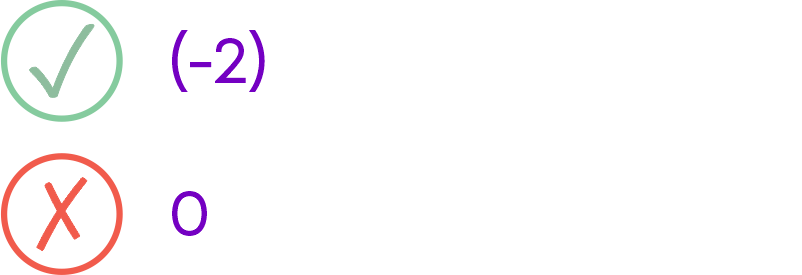#### Comparing Negative Integers

Which is greater (-98) or (-13)? Is the number with the bigger digit greater? No. For negative numbers, as the digit gets bigger, the value becomes smaller. Here, (-98) < (-13).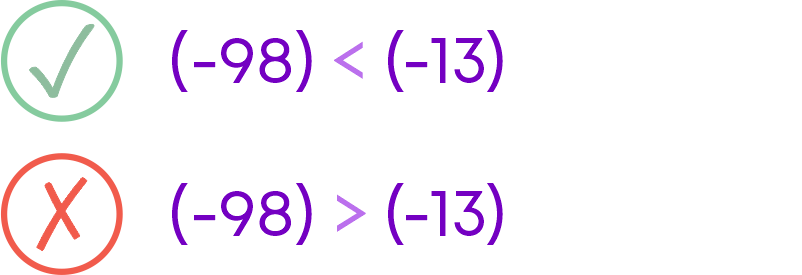• If integers are of the same sign, ADD and KEEP the SAME SIGN.
• If integers are of different signs, SUBTRACT and KEEP the SIGN of the GREATER NUMBER.

For example, let us add (-14) and (-21). Here, the two integers are of the same sign. So, we add the integers and keep the negative sign.

(-14) + (-21) = (-35)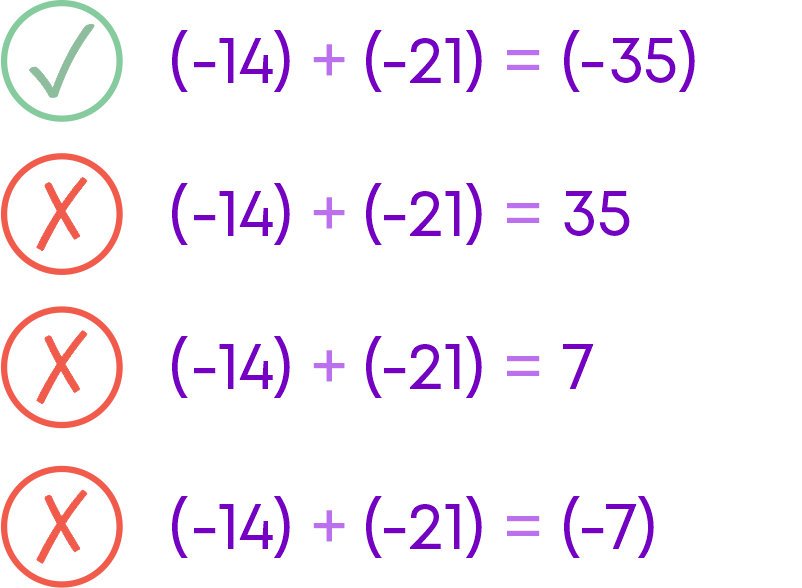Now, let us add (-14) and 21. Here, the two integers are of different signs. So, we subtract the numbers and keep the sign of the greater number.

(-14) + 21 = 7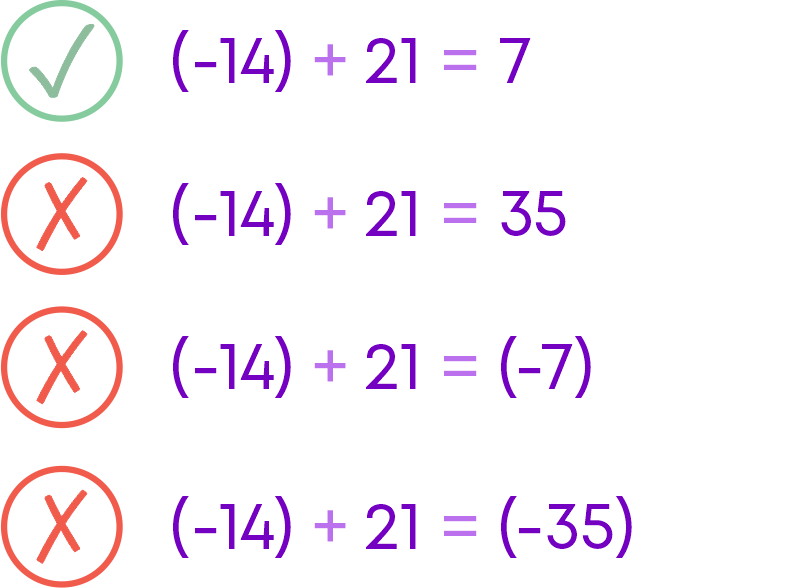#### Subtraction Of Integers

Subtraction of two integers is the same as addition with the additive inverse. For example, let us subtract 19 from 15. We need to find 15 – 19. Additive inverse of 19 is (-19).

15 – 19 = 15 + (-19) = (-4)

So, 15 – 19 = (-4)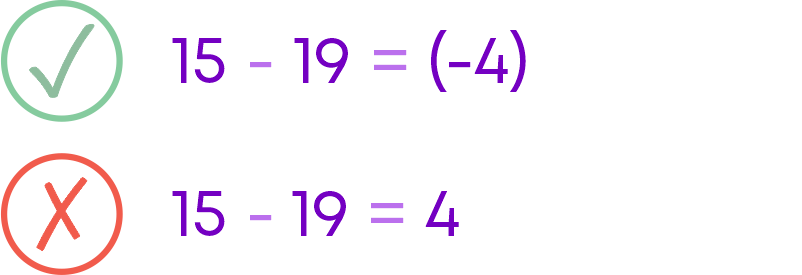Now, let us subtract (-25) from 21. We need to find 21 – (-25). Additive inverse of (-25) is 25.

21 – (-25) = 21 + 25 = 46

So, 21 – (-25) = 46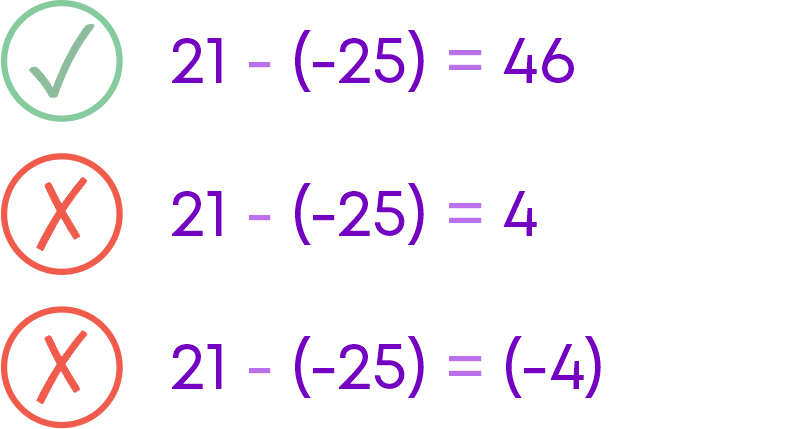Let us subtract (-25) from (-21). We need to find (-21) – (-25). Additive inverse of (-25) is 25.

(-21) – (-25) = (-21) + 25 = 4

So, (-21) – (-25) = 4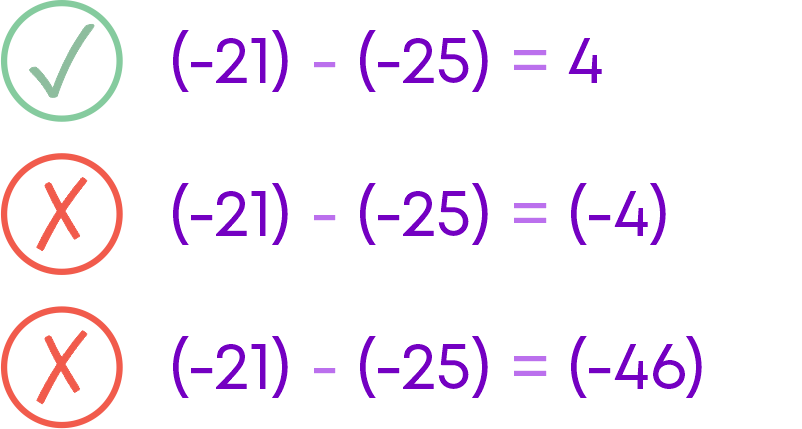Let us subtract 25 from (-21). We need to find (-21) – 25. Additive inverse of 25 is (-25).

(-21) – 25 = (-21) + (-25) = (-46)

So, (-21) – 25 = (-46)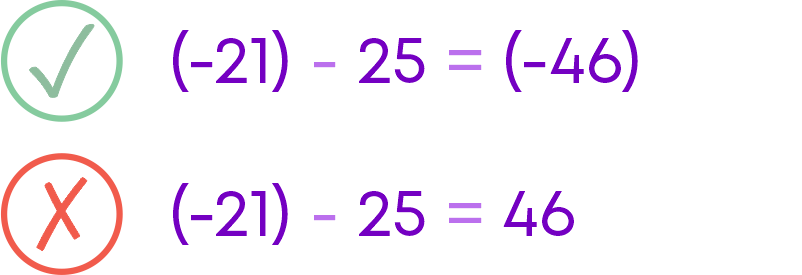#### Conclusion

We have covered all the sub-concepts in this chapter, including some common errors made by students. Can you solve this riddle based on integers?

The options below have two integers given along with some numbers. Which option contains both the square of the first integer and the cube of the second integer?

1) 2, (-3)6, 4, (-27)

2) 2, (-3)6, 4, 9

3) 3, (-2)(-4), 8, 9

4) 3, (-2)4, (-27), 8